activation_activation dense 选项 - CSDN

• 神经网络中有个词叫做 activation——激活函数现假设一神经网络N，其中w为权值参数，x为输入，b为偏置。神经网络中上层的信号 wx+b 在作为下层的输入 之前，需要 使用 激活函数激活。why？从两个角度解释A：如果不用...
神经网络中有个词叫做 activation——激活函数现假设一神经网络N，其中w为权值参数，x为输入，b为偏置。神经网络中上层的信号  wx+b 在作为下层的输入 之前，需要 使用 激活函数激活。why？从两个角度解释A：如果不用激活函数（其实相当于激活函数是f(x) = x），在这种情况下你每一层输出都是上层输入的线性函数，很容易验证，无论你神经网络有多少层，输出都是输入的线性组合，与没有隐藏层效果相当，这种情况就是最原始的感知机（Perceptron）了。引入非线性函数作为激活函数，这样深层神经网络就有意义了（不再是输入的线性组合，可以逼近任意函数）。B : 在网络N中，并不是所有的上一层信号都可以激活下一层，如果所有的上一层信号都可以激活下一层，那么这一层相当于什么都没有做。因此需要选择一些信号激活下一层的神经元。如何表示激活呢？就是当activation function的输出结果是0，就代表抑制；是1，就代表激活。Q2：为什么引入Relu呢？第一，采用sigmoid等函数，算激活函数时（指数运算），计算量大，反向传播求误差梯度时，求导涉及除法，计算量相对大，而采用Relu激活函数，整个过程的计算量节省很多。第二，对于深层网络，sigmoid函数反向传播时，很容易就会出现梯度消失的情况（在sigmoid接近饱和区时，变换太缓慢，导数趋于0，这种情况会造成信息丢失，参见@Haofeng Li答案的第三点），从而无法完成深层网络的训练。第三，Relu会使一部分神经元的输出为0，这样就造成了网络的稀疏性，并且减少了参数的相互依存关系，缓解了过拟合问题的发生（以及一些人的生物解释balabala）。当然现在也有一些对relu的改进，比如prelu，random relu等，在不同的数据集上会有一些训练速度上或者准确率上的改进，具体的大家可以找相关的paper看。多加一句，现在主流的做法，会多做一步batch normalization，尽可能保证每一层网络的输入具有相同的分布。而最新的paper，他们在加入bypass connection之后，发现改变batch normalization的位置会有更好的效果。大家有兴趣可以看下。 Ioffe S, Szegedy C. Batch normalization: Accelerating deep network training by reducing internal covariate shift[J]. arXiv preprint arXiv:1502.03167, 2015. He, Kaiming, et al. "Identity Mappings in Deep Residual Networks." arXiv preprint arXiv:1603.05027 (2016).
展开全文• 之前在使用activation function的时候只是根据自己的经验来用，例如二分类使用sigmoid或者softmax，多分类使用softmax，Dense一般都是Relu，例如tanh几乎没用过，最近在复线别人代码的时候发现别人Dense层使用的tanh...
之前在使用activation function的时候只是根据自己的经验来用，例如二分类使用sigmoid或者softmax，多分类使用softmax，Dense一般都是Relu，例如tanh几乎没用过，最近在复线别人代码的时候发现别人Dense层使用的tanh激活函数，所以决定系统的看一下各类激活函数以及其优缺点。

激活函数分类线性和非线性，例如Dense层如果不指定激活函数则会默认activation = ‘linear’，常见的非线性激活函数有sigmoid、softmax、relu、tanh等。

一、Sigmoid函数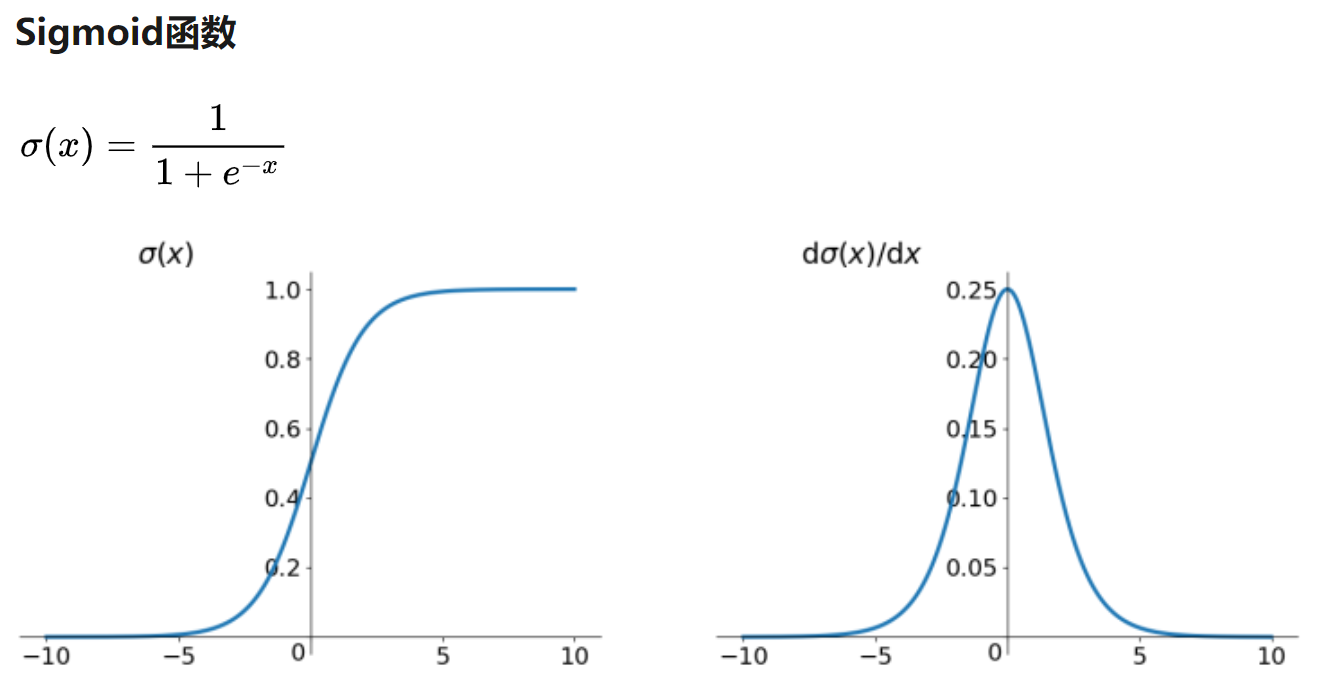sigmoid激活函数的优点是它是便于求导的平滑函数，但是缺点有：

1、容易出现梯度消散现象

2、函数的输出并不是zero-centered的

3、幂运算相对耗时

二、tanh函数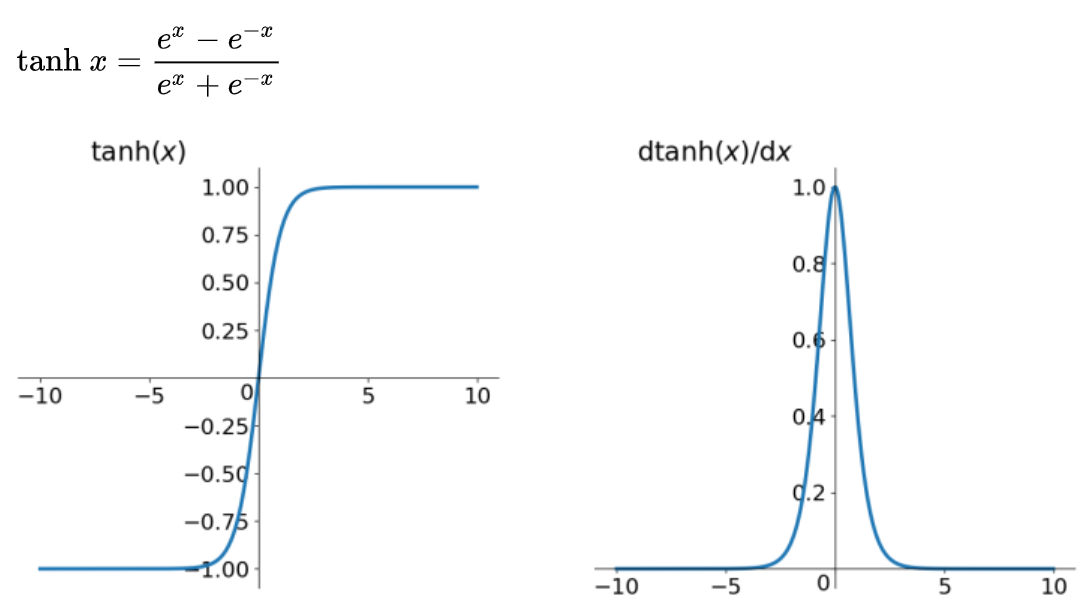tanh函数解决了zero-centered问题，但是梯度消散和幂运算问题依旧存在。

三、ReLu函数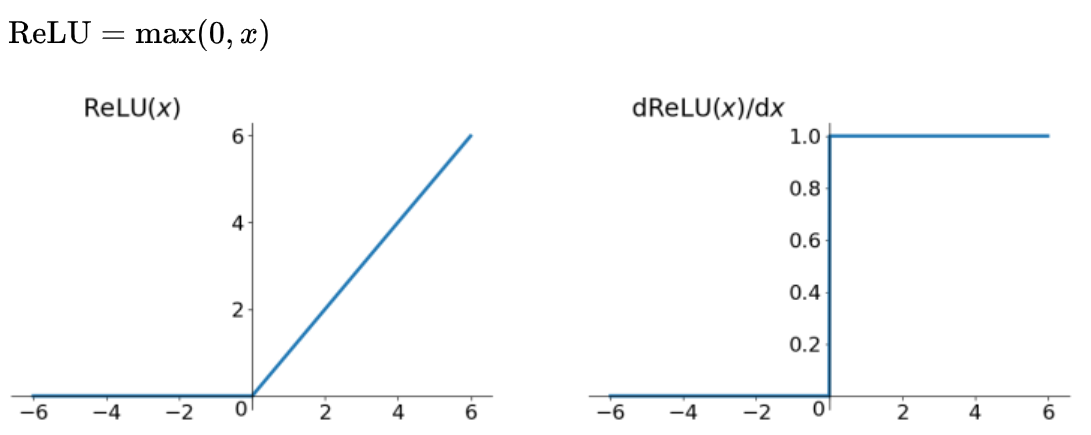ReLU函数其实就是取最大值，虽然很简单但是有很多优点：

1、解决了梯度消散的问题（在正区间）

2、计算速度快

3、收敛速度快

但是ReLU也有一些问题：

1、ReLU的输出不是zero-cemtered

2、可能会导致某些神经元永远不会被激活，从而导致对应的参数不能被更新。主要有两种可能导致这种现象的情况：(1)非常不幸的参数初始化 (2)学习率太高

但是整体来看目前最好用的还是ReLU！！！

展开全文激活函数
• 目录   激活函数 常见的激活函数 Sigmoid ...神经网络从信息处理角度对人脑神经元进行抽象，是一种运算模型，由大量的节点（神经元）之间相互连接构成。下图是一个神经元的实例图，对一个神经元来说，对输入向量x...
目录

激活函数

常见的激活函数

Sigmoid

Tanh

ReLU

Leaky ReLU

Softmax

结论

激活函数

神经网络从信息处理角度对人脑神经元进行抽象，是一种运算模型，由大量的节点（神经元）之间相互连接构成。下图是一个神经元的实例图，对一个神经元来说，对输入向量x进行加权求和，权重为W，偏置量为b，得到线性输出为，然后将求和结果z作为一个激活函数f的输入，激活函数的输出为该神经元的结果，此结果作为下一层神经元的输入。前一步的加权求和是线性变换，后一步的激活函数是非线性的。激活函数在神经网络中加入非线性使得神经网络能够更好地解决较为复杂的问题，如果缺少激活函数，一个神经网络仅仅只是一个线性的回归模型，而对于复杂的问题，神经网络将不再具有高效的学习力。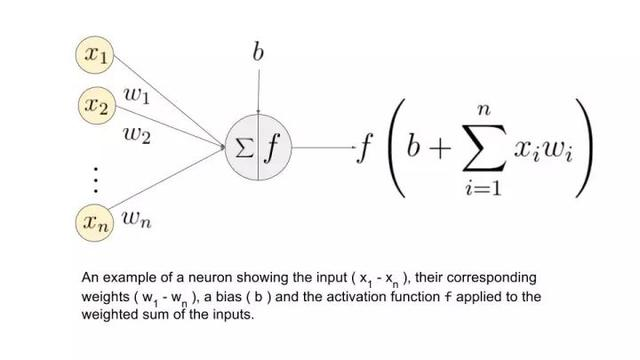常见的激活函数

激活函数的种类很多，在这里仅仅介绍一下常见的几种激活函数。

Sigmoid

Sigmoid激活函数的函数形式为，它为S型曲线，取值区间为（0,1），当x越小时，值越趋近于0，x越大的时候，值就越趋近于1，现在sigmoid激活函数很少使用，一般作为输出层的激活函数和用于二分类问题。

sigmoid激活函数的导数表达式为。下图是sigmoid原函数和其导数函数的图形。如图所示，当x>4和x<-4的时候，导数函数的取值趋近于0，使得当参数W迭代更改时，W几乎不变，使得网络很难得到有效的训练，这就是梯度消失。sigmoid激活函数需要进行指数运算，计算量大，而且反向传播求误差梯度时，很容易出现梯度消失的问题，使得不能完成深层网络的训练，除此外，sigmoid激活函数不是关于原点中心对称的。吴恩达大大在深度学习课程中说tanh激活函数几乎在任何场合的性能都优于sigmoid激活函数，除了输出层和二分类，使用sigmoid会更好点。

Tanh

tanh激活函数的数学公式为，为双曲正切函数，关于原点对称，输出区间在[-1,1]之间，使得激活函数的平均值接近于0（有类似数据中心化的效果，使得数据的平均值接近于0，能够让下一层的学习更加方便）。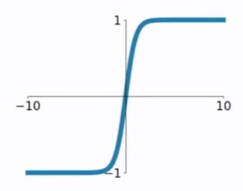tanh与sigmoid类似，可看做是由sigmoid激活函数平移得到，所以仍然存在梯度消失的问题，不过tanh的收敛速度要快于sigmoid函数。

ReLU

ReLU激活函数是Rectified linear unit的简称，是如今使用率最高的激活函数，它的数学形式为。在输入为正数的时候，输出等同于输入，在输入为负数的时候，输出为0，为分段线性函数。

当输入为正数时，其导数恒为1，不衰减，从而缓解梯度消失问题。当输入为负数时，其导数为0。由于ReLU的导数没有接近于0时减慢收敛速度的效应，所以ReLU激活函数在训练过程中会比sigmoid和tanh收敛的更快。虽然ReLU有一半的导数为0，但是在实践中，有足够多的神经元使得z大于0。

Leaky ReLU

leaky ReLU就是为了解决ReLU激活函数当x为负数时，导数为0的情况，使得当x为负数时，导数不为0，而是有一个很平缓的斜率，如下图所示。LReLU效果通常要比ReLU要好，不过实际使用的频率却没有那么高。还是ReLU更常用些。Softmax

softmax激活函数的数学形式为，用于多分类问题 ，输出每一个类别的概率大小，所有类别的概率总和为1，概率最大的类别就是输入所属的类别。

结论

sigmoid激活函数更适用于输出层，tanh几乎在所有场合都优于sigmoid，但是目前最常用的默认激活函数是ReLU
softmax更多地用于多分类问题
在建立神经网络时有很多选择，很难确定一个准则确定什么参数才更适合，所以需要在测试集或者验证集上试试各种选择，然后选择最好的。
展开全文深度学习 机器学习 激活函数
• 激活层定义typedef enum{ ...}ACTIVATION; 大家一开始看这些激活函数看起来很奇怪，下面我们会给出这几个类型的表达式CPU 端的代码激活函数对应的代码： static inline float linear_activate(float x)
激活层定义

typedef enum{
LOGISTIC, RELU, RELIE, LINEAR, RAMP, TANH, PLSE, LEAKY, ELU, LOGGY
}ACTIVATION;
大家一开始看这些激活函数看起来很奇怪，下面我们会给出这几个类型的表达式

CPU 端的代码

激活函数对应的代码：
static inline float linear_activate(float x){return x;}
static inline float logistic_activate(float x){return 1./(1. + exp(-x));}
static inline float loggy_activate(float x){return 2./(1. + exp(-x)) - 1;}
static inline float relu_activate(float x){return x*(x>0);}
static inline float elu_activate(float x){return (x >= 0)*x + (x < 0)*(exp(x)-1);}
static inline float relie_activate(float x){return x*(x>0);}
static inline float ramp_activate(float x){return x*(x>0)+.1*x;}
static inline float leaky_activate(float x){return (x>0) ? x : .1*x;}
static inline float tanh_activate(float x){return (exp(2*x)-1)/(exp(2*x)+1);}
static inline float plse_activate(float x)
{
if(x < -4) return .01 * (x + 4);
if(x > 4)  return .01 * (x - 4) + 1;
return .125*x + .5;
}
激活函数的导数：
static inline float linear_gradient(float x){return 1;}
static inline float logistic_gradient(float x){return (1-x)*x;}
{
float y = (x+1.)/2.;
return 2*(1-y)*y;
}
static inline float relu_gradient(float x){return (x>0);}
static inline float elu_gradient(float x){return (x >= 0) + (x < 0)*(x + 1);}
static inline float relie_gradient(float x){return (x>0) ? 1 : .01;}
static inline float ramp_gradient(float x){return (x>0)+.1;}
static inline float leaky_gradient(float x){return (x>0) ? 1 : .1;}
static inline float tanh_gradient(float x){return 1-x*x;}
static inline float plse_gradient(float x){return (x < 0 || x > 1) ? .01 : .125;}

GPU端的代码

//  __device__ 该函数在器件里调用，在器件中执行
//  __global__ 该函数在主机里调用，在器件中执行
//    __host__ 该函数在主机中调用，在主机中执行
激活函数对应的代码：
__device__ float linear_activate_kernel(float x){return x;}
__device__ float logistic_activate_kernel(float x){return 1./(1. + exp(-x));}
__device__ float loggy_activate_kernel(float x){return 2./(1. + exp(-x)) - 1;}
__device__ float relu_activate_kernel(float x){return x*(x>0);}
__device__ float elu_activate_kernel(float x){return (x >= 0)*x + (x < 0)*(exp(x)-1);}
__device__ float relie_activate_kernel(float x){return x*(x>0);}
__device__ float ramp_activate_kernel(float x){return x*(x>0)+.1*x;}
__device__ float leaky_activate_kernel(float x){return (x>0) ? x : .1*x;}
__device__ float tanh_activate_kernel(float x){return (exp(2*x)-1)/(exp(2*x)+1);}
__device__ float plse_activate_kernel(float x)
{
if(x < -4) return .01 * (x + 4);
if(x > 4)  return .01 * (x - 4) + 1;
return .125*x + .5;
}
激活函数的导数：
{
float y = (x+1.)/2.;
return 2*(1-y)*y;
}
__device__ float elu_gradient_kernel(float x){return (x >= 0) + (x < 0)*(x + 1);}
__device__ float relie_gradient_kernel(float x){return (x>0) ? 1 : .01;}
__device__ float leaky_gradient_kernel(float x){return (x>0) ? 1 : .1;}
__device__ float plse_gradient_kernel(float x){return (x < 0 || x > 1) ? .01 : .125;}

__device__ float activate_kernel(float x, ACTIVATION a)
{
switch(a){
case LINEAR:
return linear_activate_kernel(x);
case LOGISTIC:
return logistic_activate_kernel(x);
case LOGGY:
return loggy_activate_kernel(x);
case RELU:
return relu_activate_kernel(x);
case ELU:
return elu_activate_kernel(x);
case RELIE:
return relie_activate_kernel(x);
case RAMP:
return ramp_activate_kernel(x);
case LEAKY:
return leaky_activate_kernel(x);
case TANH:
return tanh_activate_kernel(x);
case PLSE:
return plse_activate_kernel(x);
}
return 0;
}
__device__ float gradient_kernel(float x, ACTIVATION a)
{
switch(a){
case LINEAR:
case LOGISTIC:
case LOGGY:
case RELU:
case ELU:
case RELIE:
case RAMP:
case LEAKY:
case TANH:
case PLSE:
}
return 0;
}

__global__ void activate_array_kernel(float *x, int n, ACTIVATION a)
{
int i = (blockIdx.x + blockIdx.y*gridDim.x) * blockDim.x + threadIdx.x;
if(i < n) x[i] = activate_kernel(x[i], a);
}

__global__ void gradient_array_kernel(float *x, int n, ACTIVATION a, float *delta)
{
int i = (blockIdx.x + blockIdx.y*gridDim.x) * blockDim.x + threadIdx.x;
if(i < n) delta[i] *= gradient_kernel(x[i], a);
}
//extern "C"表明了一种编译规约，其中extern是关键字属性，“C”表征了编译器链接规范。对于extern "C"可以理解成在C++/C中的混合编程的编译指令。
extern "C" void activate_array_ongpu(float *x, int n, ACTIVATION a)
{
activate_array_kernel<<<cuda_gridsize(n), BLOCK>>>(x, n, a);
check_error(cudaPeekAtLastError());
}

extern "C" void gradient_array_ongpu(float *x, int n, ACTIVATION a, float *delta)
{
check_error(cudaPeekAtLastError());
}
//这个地方已经定义了BLOCK的大小为512，这个函数的功能是使得设置网格的维度，要求是x的大小不超过65535，返回网格的大小。网格的示意图如下图。
dim3 cuda_gridsize(size_t n){
size_t k = (n-1) / BLOCK + 1;
size_t x = k;
size_t y = 1;
if(x > 65535){
x = ceil(sqrt(k));
y = (n-1)/(x*BLOCK) + 1;
}
dim3 d = {x, y, 1};
return d;
}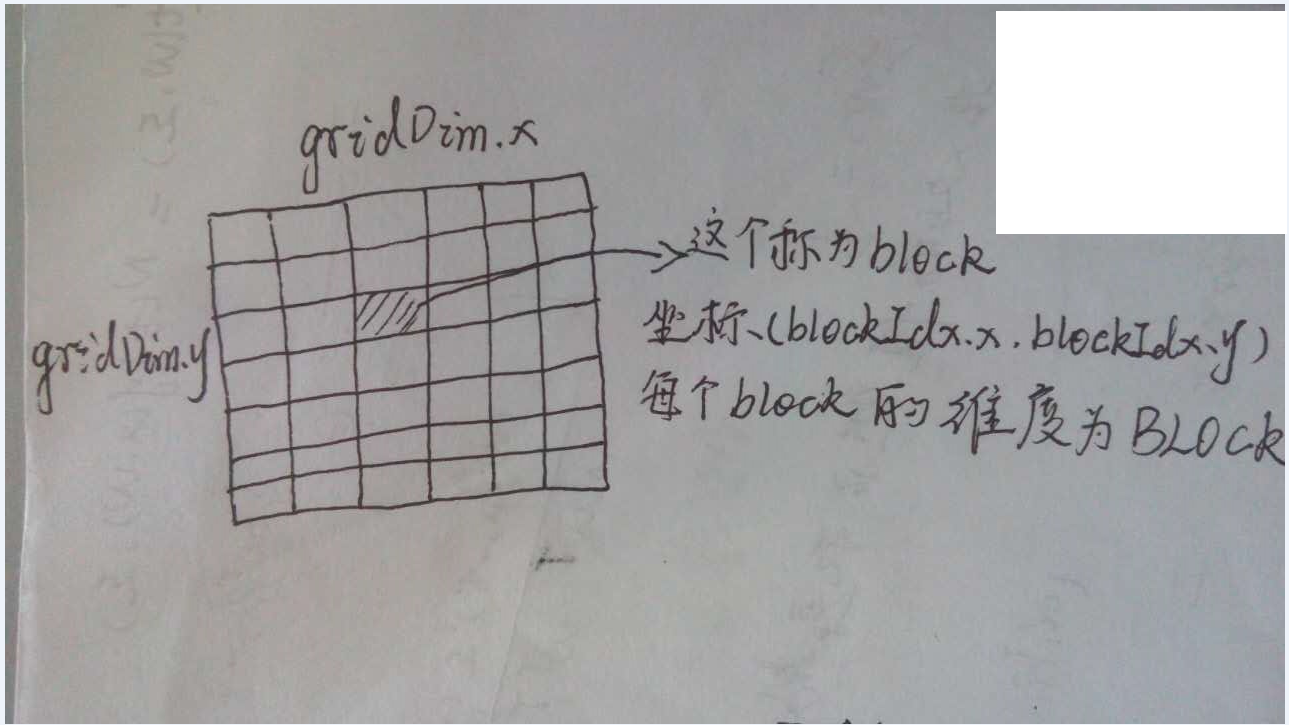对于给定的线程的坐标为（blockIdx.x,blockIdx.y,threadIdx.x,threadIdx.y）

对应于这个程序代码里面 ，BlockDim.x = BLOCK , BlockDim.y = 1, threadIdx.y = 0
对应的线程标号为 (blockIdx.y*gridDim.x + blockIdx.x)*BlockDim.x* + threadIdx.y

LOGISTIC 的表达式

y=1.01.0+e−x<!--//--><![CDATA[//><!--
y = \frac{1.0}{1.0 + e^{-x}}
//--><!]]>dydx=1<!--//--><![CDATA[//><!--
\frac{dy}{dx} = 1
//--><!]]>RELU 的表达式

y={x0 if x>0 if x≤0<!--//--><![CDATA[//><!--
y=\begin{cases}
x & \text{ if	} x\gt 0  \\
0 & \text{ if	} x \leq 0
\end{cases}
//--><!]]>RELIE 的表达式

y={x0if x>0if x≤0<!--//--><![CDATA[//><!--
y =\begin{cases}
x & \text {if	} x \gt 0 \\
0 & \text {if	} x \leq 0
\end{cases}
//--><!]]>dydx={10if x>0if x≤0<!--//--><![CDATA[//><!--
\frac{dy}{dx} = \begin{cases}
1 & \text {if } x \gt 0 \\
0 & \text{if } x \leq 0
\end{cases}
//--><!]]>LINEAR 的表达式

y=x<!--//--><![CDATA[//><!--
y = x
//--><!]]>dydx=1<!--//--><![CDATA[//><!--
\frac{dy}{dx} = 1
//--><!]]>RAMP 的表达式

y={x+0.1x0.1xif x>0if x≤0<!--//--><![CDATA[//><!--
y =\begin{cases}
x + 0.1 x & \text {if	} x \gt 0 \\
0.1x & \text {if	} x \leq 0
\end{cases}
//--><!]]>dydx={1+0.10.1if x>0if x≤0<!--//--><![CDATA[//><!--
\frac{dy}{dx} = \begin{cases}
1 + 0.1  & \text {if	} x \gt 0 \\
0.1 & \text {if	} x \leq 0
\end{cases}
//--><!]]>TANH 的表达式

y=e2x−1e2x+1<!--//--><![CDATA[//><!--
y = \frac{e^{2x} - 1}{e^{2x} + 1}
//--><!]]>dydx=1−x2<!--//--><![CDATA[//><!--
\frac{dy}{dx} = 1 - x^{2}
//--><!]]>PLSE 的表达式

y=⎧⎩⎨0.01(x+4)0.01(x−4)0.125x+0.5if x<−4if x>4if x=4<!--//--><![CDATA[//><!--
y =\begin{cases}
0.01(x+4)& \text {if	} x \lt -4 \\
0.01(x-4) & \text {if	} x \gt 4 \\
0.125x + 0.5& \text {if } x  = 4
\end{cases}
//--><!]]>dydx={0.010.125if x<0∥x>1if x=4<!--//--><![CDATA[//><!--
\frac{dy}{dx} = \begin{cases}
0.01& \text {if	} x \lt 0  \| x \gt 1 \\
0.125& \text {if } x  = 4
\end{cases}
//--><!]]>LEAKY 的表达式

y={x0.1xif x>0if x≤0<!--//--><![CDATA[//><!--
y =\begin{cases}
x& \text {if	} x \gt 0 \\
0.1x& \text {if	} x \leq 0
\end{cases}
//--><!]]>dydx={10.1if x>0if x≤0<!--//--><![CDATA[//><!--
\frac{dy}{dx}=\begin{cases}
1& \text {if	} x \gt 0 \\
0.1& \text {if	} x \leq 0
\end{cases}
//--><!]]>ELU 的表达式

y={xex−1if x≥0if x<0<!--//--><![CDATA[//><!--
y =\begin{cases}
x& \text {if	} x \geq 0 \\
e^{x} - 1& \text {if	} x \lt 0
\end{cases}
//--><!]]>dydx={1x+1if x≥0if x<0<!--//--><![CDATA[//><!--
\frac{dy}{dx} =\begin{cases}
1& \text {if	} x \geq 0 \\
x+1& \text {if	} x \lt 0
\end{cases}
//--><!]]>LOGGY 的表达式

y=2.01.0+e−x−1=1.0−e−x1.0+e−x<!--//--><![CDATA[//><!--
y =\frac{2.0}{1.0 + e^{-x}} - 1 = \frac{1.0 - e^{-x}}{1.0 + e^{-x}}
//--><!]]>dydx=21−x1+x<!--//--><![CDATA[//><!--
\frac{dy}{dx} =2\frac{1-x}{1+x}
//--><!]]>
展开全文YOLO c
• activation\activation\1.0.2 解决maven 无法下载问题 将文件拷贝到目录本地maven 库 即可
• ## CAM（Class Activation Mapping）通俗篇

万次阅读 多人点赞 2020-10-10 15:37:26
同时还能够针对图片中的关键部分进行定位，这个过程被称为Class Activation Mapping，简称CAM。 那么什么叫做CAM呢，如下图所示，第一组输入图片，训练的标签式刷牙，那么通过based model+CA...GAP CAM
• activation-1.1.jar写JAVAweb项目时用到邮箱相关操作会用到开发
• 1.获取注册码 进入该网页：http://idea.lanyus.com/ 获得注册码 2.填写注册码 将获得的注册码填入输入框里OK
• https://blog.csdn.net/a107473515/article/details/85167818https://blog.csdn.net/lw545034502/article/details/85935427
• CentOS的启动出错：当执行 ifup ens33出现错误：Error:Connection activation failed: No suitable device found for this connection解决办法：chkconfig NetworkManager offchkconfig network onservice ...Centos
• 用别人的破解文件双击时，ACTIVATION_KEY 老是为null 解决办法：以管理员身份运行命令行，进入到破解工具的文件夹，运行run.bat（命令行下直接 run 即可）。就会成功生成正确的ACTIVATION_KEY
• 今天打开vm 发现建完的三台虚拟机居然没法联通了，emmm这俩 会自己关掉。。。
• 1、右键【MyEclipse CI】，然后点击【显示包内容】 2、依次进入Contents--&gt;Profile--&gt;plugins   3、将com.genuitec.eclipse.core_16.0.0.201808301917.jar文件改名 4、选择Blue版本后点击右...Myeclipse
• softmax：对输入数据的最后一维进行softmax，输入数据应形如(nb_samples, nb_timesteps, nb_dims)或(nb_samples,nb_dims)eluselu: 可伸缩的指数线性单元（Scaled Exponential Linear Unit），参考Self-Normalizing ...
• Linux Centos 6.5网络启动不起来Bringing up interface eth0: Error:Connection activation failed:Device not managed by NetworkManager
• 2019最新版IDEA 用lanyus注册码激活时出现 Your activation code could not be validated （error 1653219） 按照lanyus官网提示必须添加“0.0.0.0 account.jetbrains.com”及“0.0.0.0 www.jetbrains.com”到hosts...idea激活
• 缺少activation-1.0.2.jar错误提示java.lang.NoClassDefFoundError: javax/activation/FileTypeMap
• 参考上述链接的破解教程时到了第三步： ...在此再次启动WebStorm，选择activation code，并将-javaagent:你的WebStorm路径前缀/WebStorm/bin/破解补丁名字.jar 时，由于正在试用期，找不到开始进入时的act...webstorm 前端
• Android发邮件时出现一下...04-20 09:28:23.663: E/AndroidRuntime(13202): java.lang.NoClassDefFoundError: javax.activation.DataHandler 04-20 09:28:23.663: E/AndroidRuntime(13202): at javax.mail.internet.MiAndroid
• VMware中安装的centos6.6 64位，重启...Bringing up interface eth0: Error:Connection activation failed:Device not managed by NetworkManager 解决方法： 1、Remove Network Manager from startup Servic网络 Linux
...